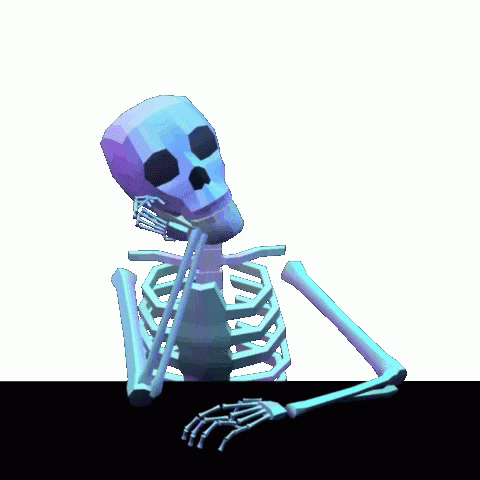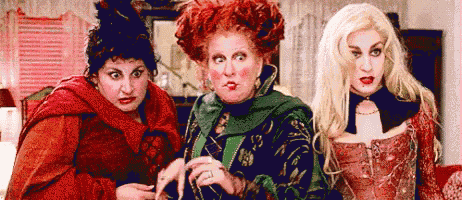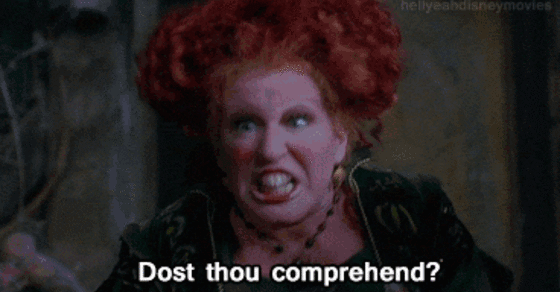Holiday & Seasonal

# This Halloween Math Puzzle Is Stumping People Online

### Can you solve it?

Halloween is scary, what with all the costumes and the ghosts and the amount of sugar in candy corn. Do you know what else is scary? Algebra, what with all the numbers and the rules and the math.

So a Halloween-themed riddle seems like a little much. But much like a haunted house, we shall conquer our fear and march onward. Here is the problem from Mathway that was shared on Oct. 20, and is stumping people on the Internet. Are you ready to give it a whirl?

Spooky enough for you? We’ll wait here while you try and solve this spooky math problem. While we’re young…Have your answer? Well, flip the page to see if got a trick or a treat.Not seeing how we got to that number? Are you getting 20? Well, take a closer look at the bats in the equation. Notice a difference between the bats in the third line and the bats in the fourth line?

That’s right, there are only two bats in the fourth line. By this logic, the problem is treating each bat as a symbol, rather than all of the bats together being a unit. With that in mind, let’s give it another try.

So each ghost is 4, because 4 + 4 + 4 = 12.

Then, each pumpkin is worth 8, because 4 + 8 + 8 = 20.

And then, each bat is worth 1, because 8 + (1+1+1) + (1+1+1) = 14.

So the final equation is 8 + 4 x (1+1)= ?

Remembering Dear Aunt Sally and the Order of Operations (parentheses, exponents, multiplication, division, addition, subtraction) you get:

8 + 4 x 2 = 8 + 8 = 16.And there you have it, folks. Want to try that again, but with a different theme? Well, Mathway has a back-to-school one, a breakfast one, a sports bar one, a fall one and … yeah, you get the point.

If you want to try your hand at more brain-teasing puzzles, check out this algebra puzzle or this train problem.